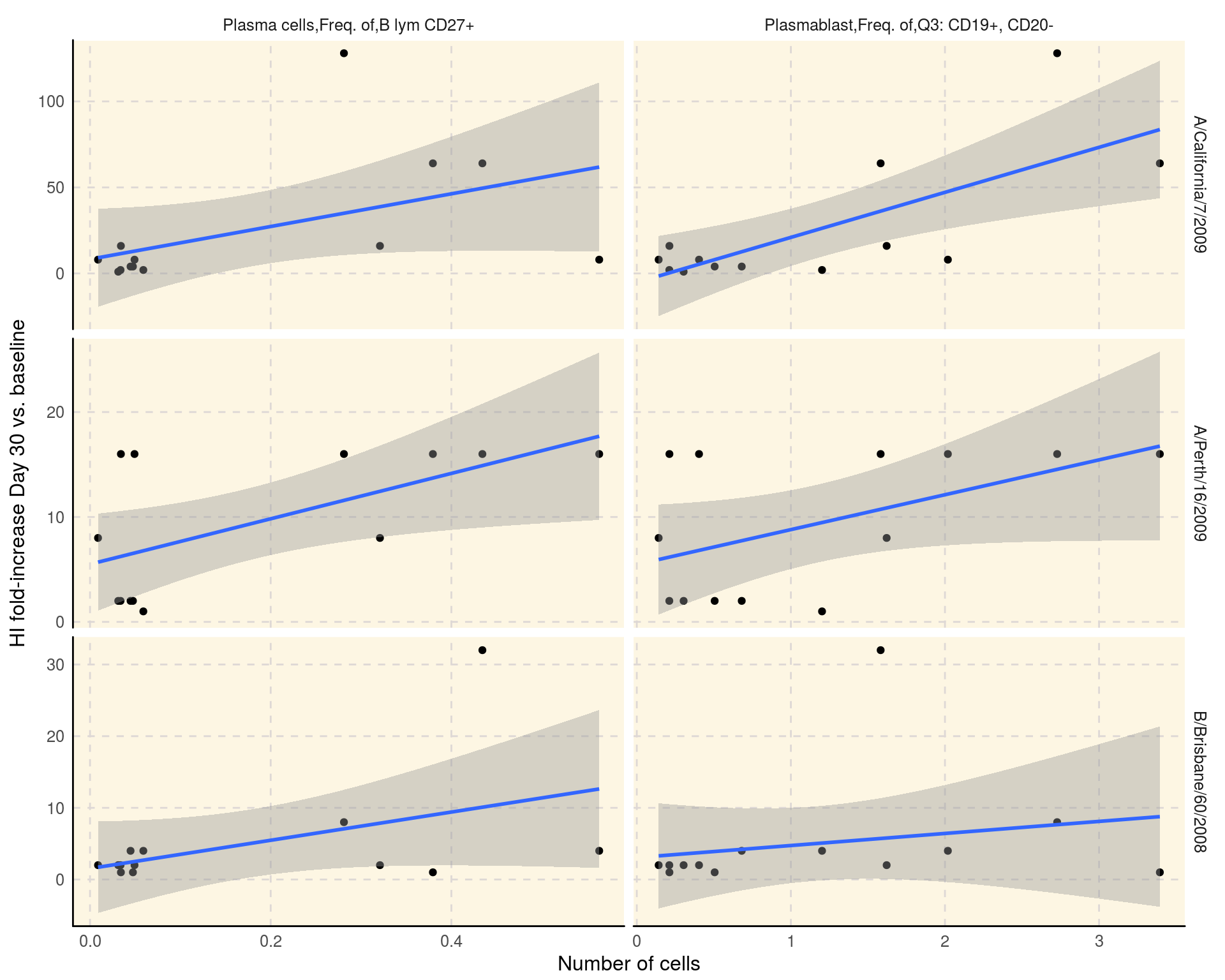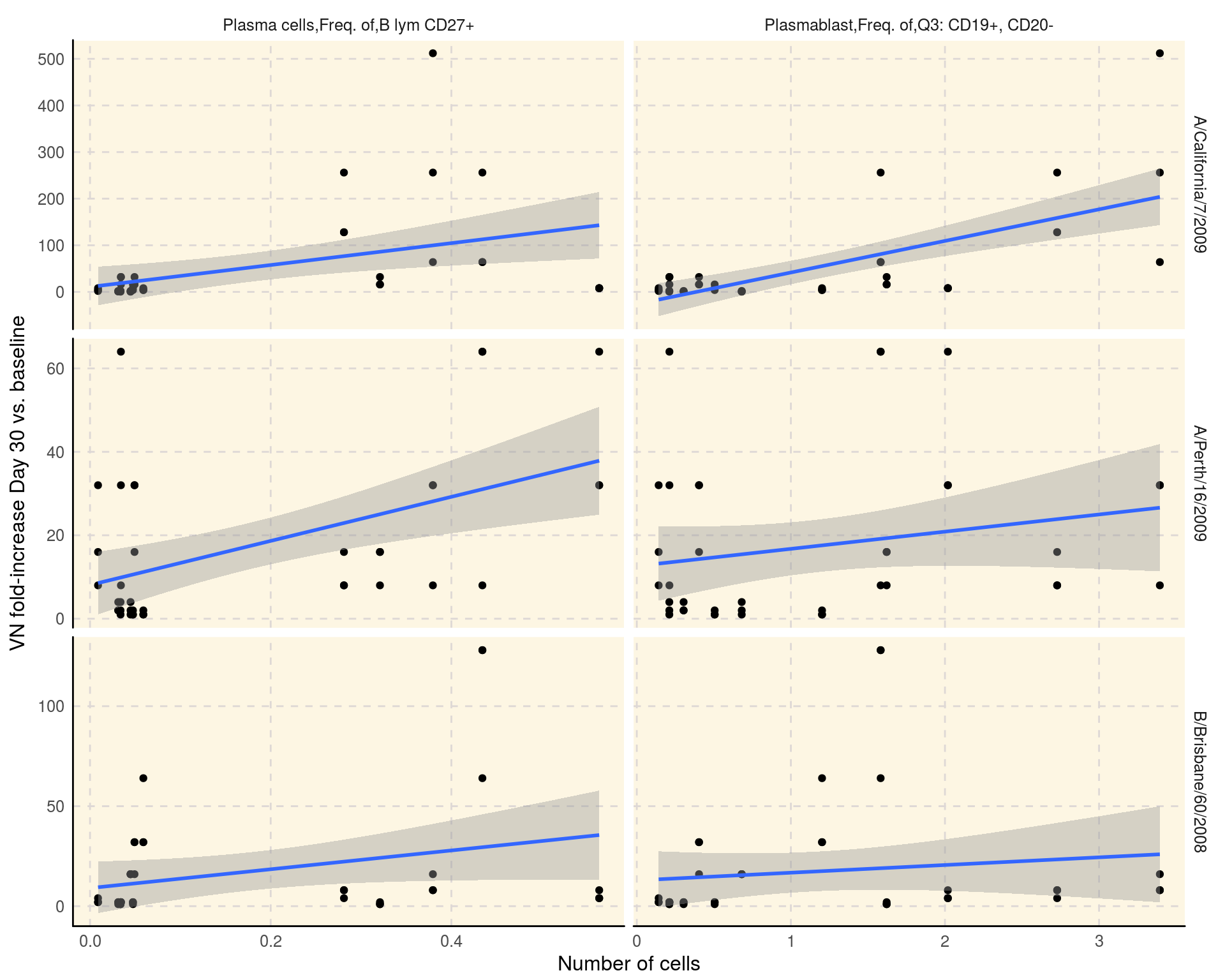# Correlation of HAI/virus neutralizition titer and cell counts in SDY144

### Correlations between hemagglutination inhibition (HI) and viral neutralization (VN) titers and plasmablast and plasma B cells among trivalent inactivated influenza vaccine (TIV) vaccinees.

This reports reproduces Figure 2 of Cao RG et al(2014) published as part of the original study.

library(ImmuneSpaceR)
library(data.table)
library(ggplot2)

#### Initialize the connection to SDY144 and get data

First we initialize the connection to the selected study using CreateConnection. Then we grab the datasets of interests with the getDataset method.

con <- CreateConnection("SDY144")
flow <- con$getDataset("fcs_analyzed_result", maxRows = 10000) # maxRows for Rlabkey 2.1.136 / LK 17.2 hai <- con$getDataset("hai")
vn   <- con\$getDataset("neut_ab_titer")

Then we select the cell populations and time points of intereset.

pb <- flow[population_name_reported %in% c("Plasma cells,Freq. of,B lym CD27+",
"Plasmablast,Freq. of,Q3: CD19+, CD20-")]
pb <- pb[, population_cell_number := as.numeric(population_cell_number)]
pb <- pb[study_time_collected == 7 & study_time_collected_unit == "Days"] #13 subjects
pb <- pb[, list(participant_id, population_cell_number, population_name_reported)]

We compute the HI and VN titer as the fold-increase between baseline and day 30.

# HAI
hai <- hai[,response:=value_reported/value_reported[study_time_collected==0],
by="virus,cohort,participant_id"][study_time_collected==30]
hai <- hai[, list(participant_id, virus, response)]
dat_hai <- merge(hai, pb, by = "participant_id", allow.cartesian = TRUE)
# VN
vn <- vn[, response:=value_reported/value_reported[study_time_collected==0],
by="virus,cohort,participant_id"][study_time_collected==30]
vn <- vn[, list(participant_id, virus, response)]
dat_vn <- merge(vn, pb, by = "participant_id", allow.cartesian = TRUE)

#### Plot using ggplot2

Figure 2 A: Correlation between the absolute number of plasmablasts and plasma Bcells 7 days after vaccination with and fold-increase of HI titers from baseline to day 30 after vaccination.

ggplot(dat_hai, aes(x = population_cell_number, y = response)) +
geom_point() + geom_smooth(method = "lm") +
facet_grid(virus~population_name_reported, scale = "free") +
xlab("Number of cells") + ylab("HI fold-increase Day 30 vs. baseline") + theme_IS()Figure 2 B: Correlation between the absolute number of plasmablasts and plasma Bcells 7 days after vaccination with and fold-increase of VN titers from baseline to day 30 after vaccination.

ggplot(dat_vn, aes(x = population_cell_number, y = response)) +
geom_point() + geom_smooth(method = "lm") +
facet_grid(virus~population_name_reported, scale = "free") +
xlab("Number of cells") + ylab("VN fold-increase Day 30 vs. baseline") + theme_IS()